International
Tables for
Crystallography
Volume E
Subperiodic groups
Edited by V. Kopský and D. B. Litvin

International Tables for Crystallography (2006). Vol. E, ch. 1.2, pp. 5-7   | 1 | 2 |

## Section 1.2.1. Classification of subperiodic groups

V. Kopskýa and D. B. Litvinb*

aDepartment of Physics, University of the South Pacific, Suva, Fiji, and Institute of Physics, The Academy of Sciences of the Czech Republic, Na Slovance 2, PO Box 24, 180 40 Prague 8, Czech Republic, and bDepartment of Physics, Penn State Berks Campus, The Pennsylvania State University, PO Box 7009, Reading, PA 19610-6009, USA
Correspondence e-mail:  u3c@psu.edu

### 1.2.1. Classification of subperiodic groups

| top | pdf |

Subperiodic groups can be classified in ways analogous to the space groups. For the mathematical definitions of these classifications and their use for space groups, see Chapter 8.2of IT A (2005). Here we shall limit ourselves to those classifications which are explicitly used in the symmetry tables of the subperiodic groups.

#### 1.2.1.1. Subperiodic group types

| top | pdf |

The subperiodic groups are classified into affine subperiodic group types, i.e. affine equivalence classes of subperiodic groups. There are 80 affine layer-group types and seven affine frieze-group types. There are 67 crystallographic and an infinity of noncrystallographic affine rod-group types. We shall consider here only rod groups of the 67 crystallographic rod-group types and refer to these crystallographic affine rod-group types simply as affine rod-group types.

The subperiodic groups are also classified into proper affine subperiodic group types, i.e. proper affine classes of subperiodic groups. For layer and frieze groups, the two classifications are identical. For rod groups, each of eight affine rod-group types splits into a pair of enantiomorphic crystallographic rod-group types. Consequently, there are 75 proper affine rod-group types. The eight pairs of enantiomorphic rod-group types are(R24),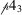(R26);(R31),(R33);(R43),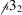(R44);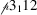(R47),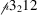(R48);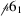(R54),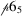(R58);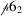(R55),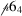(R57);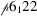(R63),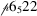(R67); and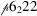(R64),(R66). (Each subperiodic group is given in the text by its Hermann–Mauguin symbol followed in parenthesis by a letter L, R or F to denote it, respectively, as a layer, rod or frieze group, and its sequential numbering from Parts 2, 3or 4.) We shall refer to the proper affine subperiodic group types simply as subperiodic group types.

#### 1.2.1.2. Other classifications

| top | pdf |

There are 27 geometric crystal classes of layer groups and rod groups, and four geometric crystal classes of frieze groups. These are listed, for layer groups, in the fourth column of Table 1.2.1.1, and for the rod and frieze groups in the second columns of Tables 1.2.1.2and 1.2.1.3, respectively.

 Table 1.2.1.1| top | pdf | Classification of layer groups
 Bold or bold underlined symbols indicate Laue groups. Bold underlined point groups are also lattice point symmetries (holohedries).
Two-dimensional Bravais systemSymbolThree-dimensional crystal systemCrystallographic point groupsNo. of layer-group typesRestrictions on conventional coordinate systemCell parameters to be determinedBravais lattice
Oblique m Triclinic 1,2 None a, b, γ mp
Monoclinic 2, m, 2/m 5 α = β = 90°
Rectangular o 11 β = γ = 90° a, b op
Orthorhombic 222, 2mm, mmm 30 α = β = γ = 90° oc
Square t Tetragonal 4,, 4/m 16 a = b a tp
422, 4mm,2m, 4/mmm α = β = γ = 90°
Hexagonal h Trigonal 3,8 a = b a hp
32, 3m,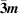Hexagonal 6,, 6/m 8 γ = 120°
622, 6mm,m2, 6/mmm α = β = 90°
This angle is conventionally taken to be non-acute, i.e.90°.
 Table 1.2.1.2| top | pdf | Classification of rod groups
 Bold symbols indicate Laue groups.
Three-dimensional crystal system Crystallographic point groups No. of rod-group types Restrictions on conventional coordinate system
Triclinic 1,2 None
Monoclinic (inclined) 2, m, 2/m 5 β = γ = 90°
Monoclinic (orthogonal) 5 α = β = 90°
Orthorhombic 222, 2mm, mmm 10 α = β = γ = 90°
Tetragonal 4,, 4/m 19
422, 4mm,2m, 4/mmm
Trigonal 3,11 α = β = 90, γ = 120°
32, 3m,m
Hexagonal 6,, 6/m 23
622, 6mm,m2, 6/mmm
 Table 1.2.1.3| top | pdf | Classification of frieze groups
 Bold symbols indicate Laue groups.
Two-dimensional crystal systemCrystallographic point groupsNo. of frieze-group typesRestrictions on conventional coordinate system
Oblique 1, 2 2 None
Rectangular m, 2mm 5 γ = 90°

We further classify subperiodic groups according to the following classifications of the subperiodic group's point group and lattice group. These classifications are introduced to emphasize the relationships between subperiodic groups and space groups:

 (1) The point group of a layer or rod group is three-dimensional and corresponds to a point group of a three-dimensional space group. The point groups of three-dimensional space groups are classified into the triclinic, monoclinic, orthorhombic, tetragonal, trigonal, hexagonal and cubic crystal systems. We shall use this classification also for subperiodic groups. Consequently, the three-dimensional subperiodic groups are classified, see the third column of Table 1.2.1.1and the first column of Table 1.2.1.2, into the triclinic, monoclinic, orthorhombic, tetragonal, trigonal and hexagonal crystal systems. The cubic crystal system does not arise for three-dimensional subperiodic groups. Two-dimensional subperiodic groups, i.e. frieze groups, are analogously classified, see the first column of Table 1.2.1.3, into the oblique and rectangular crystal systems. (2) The two-dimensional lattice of a layer group is also a two-dimensional lattice of a plane group. The lattices of plane groups are classified, according to Bravais (flock) systems, see IT A (2005), into the oblique, rectangular, square and hexagonal Bravais systems. We shall also use this classification for layer groups, see the first column in Table 1.2.1.1. For rod and frieze groups no lattice classification is used, as all one-dimensional lattices form a single Bravais system.

A subdivision of the monoclinic rod-group category is made into monoclinic/inclined and monoclinic/orthogonal. Two different coordinate systems, see Table 1.2.1.2, are used for the rod groups of these two subdivisions of the monoclinic crystal system. These two coordinate systems differ in the orientation of the plane containing the non-lattice basis vectors relative to the lattice vectors. For the monoclinic/inclined subdivision, the plane containing the non-lattice basis vectors is, see Fig. 1.2.1.1, inclined with respect to the lattice basis vector. For the monoclinic/orthogonal subdivision, the plane is, see Fig. 1.2.1.2, orthogonal.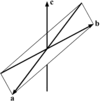Figure 1.2.1.1 | top | pdf |Monoclinic/inclined basis vectors. For the monoclinic/inclined subdivision, β = γ = 90° and the plane containing the a and b non-lattice basis vectors is inclined with respect to the lattice basis vector c.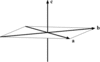Figure 1.2.1.2 | top | pdf |Monoclinic/orthogonal basis vectors. For the monoclinic/orthogonal subdivision, α = β = 90° and the plane containing the a and b non-lattice basis vectors is orthogonal to the lattice basis vector c.

#### 1.2.1.2.1. Conventional coordinate systems

| top | pdf |

The subperiodic groups are described by means of a crystallographic coordinate system consisting of a crystallographic origin, denoted by O, and a crystallographic basis. The basis vectors for the three-dimensional layer groups and rod groups are labelled a, b and c. The basis vectors for the two-dimensional frieze groups are labelled a and b. Unlike space groups, not all basis vectors of the crystallographic basis are lattice vectors. Like space groups, the crystallographic coordinate system is used to define the symmetry operations (see Section 1.2.9) and the Wyckoff positions (see Section 1.2.11). The symmetry operations are defined with respect to the directions of both lattice and non-lattice basis vectors. A Wyckoff position, denoted by a coordinate triplet (x, y, z) for the three-dimensional layer and rod groups, is defined in the crystallographic coordinate system by O + r, where r = xa + yb + zc. For the two-dimensional frieze groups, a Wyckoff position is denoted by a coordinate doublet (x, y) and is defined in the crystallographic coordinate system by O + r, where r = xa + yb.

The term setting will refer to the assignment of the labels a, b and c (and the corresponding directions ,  and , respectively) to the basis vectors of the crystallographic basis (see Section 1.2.6). In the standard setting, those basis vectors which are also lattice vectors are labelled as follows: for layer groups with their two-dimensional lattice by a and b, for rod groups with their one-dimensional lattice by c, and for frieze groups with their one-dimensional lattice by a.

The selection of a crystallographic coordinate system is not unique. Following IT A (2005), we choose conventional crystallographic coordinate systems which have a right-handed set of basis vectors and such that symmetry of the subperiodic groups is best displayed. The conventional crystallographic coordinate systems used in the standard settings are given in the sixth column of Table 1.2.1.1for the layer groups, and the fourth columns of Tables 1.2.1.2and 1.2.1.3for the rod groups and frieze groups, respectively. The crystallographic origin is conventionally chosen at a centre of symmetry or at a point of high site symmetry (see Section 1.2.7).

The conventional unit cell of a subperiodic group is defined by the crystallographic origin and by those basis vectors which are also lattice vectors. For layer groups in the standard setting, the cell parameters, the magnitude of the lattice basis vectors a and b, and the angle between them, which specify the conventional cell, are given in the seventh column of Table 1.2.1.1. The conventional unit cell obtained in this manner turns out to be either primitive or centred and is denoted by p or c, respectively, in the eighth column of Table 1.2.1.1. For rod and frieze groups with their one-dimensional lattices, the single cell parameter to be specified is the magnitude of the lattice basis vector.

### References

International Tables for Crystallography (2005). Vol. A. Space-group symmetry, edited by Th. Hahn. Heidelberg: Springer. [Previous editions: 1983, 1987, 1992, 1995 and 2002. Abbreviated as IT A (2005).]Google Scholar